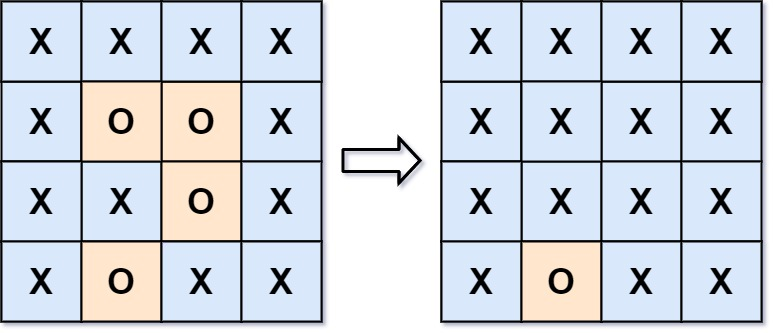0130. 被围绕的区域

# 0130. 被围绕的区域#

• 标签：深度优先搜索、广度优先搜索、并查集、数组、矩阵
• 难度：中等

## 题目大意 #

• $m == board.length$。
• $n == board[i].length$。
• $1 <= m, n <= 200$。
• $board[i][j]$ 为 'X''O'

• 示例 1：1 2 3  输入：board = [["X","X","X","X"],["X","O","O","X"],["X","X","O","X"],["X","O","X","X"]] 输出：[["X","X","X","X"],["X","X","X","X"],["X","X","X","X"],["X","O","X","X"]] 解释：被围绕的区间不会存在于边界上，换句话说，任何边界上的 'O' 都不会被填充为 'X'。 任何不在边界上，或不与边界上的 'O' 相连的 'O' 最终都会被填充为 'X'。如果两个元素在水平或垂直方向相邻，则称它们是“相连”的。 
• 示例 2：
 1 2  输入：board = [["X"]] 输出：[["X"]] 

## 解题思路 #

### 思路 1：代码 #

  1 2 3 4 5 6 7 8 9 10 11 12 13 14 15 16 17 18 19 20 21 22 23 24 25 26 27 28 29 30 31 32  class Solution: def solve(self, board: List[List[str]]) -> None: """ Do not return anything, modify board in-place instead. """ if not board: return rows, cols = len(board), len(board) def dfs(x, y): if not 0 <= x < rows or not 0 <= y < cols or board[x][y] != 'O': return board[x][y] = '#' dfs(x + 1, y) dfs(x - 1, y) dfs(x, y + 1) dfs(x, y - 1) for i in range(rows): dfs(i, 0) dfs(i, cols - 1) for j in range(cols - 1): dfs(0, j) dfs(rows - 1, j) for i in range(rows): for j in range(cols): if board[i][j] == '#': board[i][j] = 'O' elif board[i][j] == 'O': board[i][j] = 'X' 

### 思路 1：复杂度分析 #

• 时间复杂度：$O(n \times m)$，其中 $m$ 和 $n$ 分别为行数和列数。
• 空间复杂度：$O(n \times m)$。